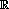Surfaces

Start this free course now. Just create an account and sign in. Enrol and complete the course for a free statement of participation or digital badge if available.

Free course

# 4.4 Historical note on the Euler characteristic

A little history is instructive here, because it shows how difficult Theorem 9 really is. By 1900 the classification of compact surfaces was well understood, although proofs of the major theorems relied more on intuition than would be acceptable today. Attention switched to objects called ‘3-manifolds’, topological spaces each point of which has a neighbourhood homeomorphic to the interior of the unit ball in3. The French mathematician Henri Poincaré – one of the leading mathematicians of his time – was among the first to study spaces of three or more dimensions. He proposed to study 3-manifolds by triangulating them – that is, by subdividing them into tetrahedra. Faced with the three-dimensional version of the problem of finding properties of a 3-manifold that are independent of any triangulation, he conjectured that given any two triangulations, there is a further triangulation which contains both. But not even he could prove it. His conjecture stood until 1948, and the corresponding result for2 was proved only in 1963.

Perhaps the most eloquent indication of the difficulty of the proof of Theorem 9 was the remarkable discovery (by the American mathematician John Milnor in 1961) that the corresponding conjecture is false for spaces of dimension 6: this means that any proof in three dimensions must use some special property of three-dimensional space. This was one of a number of achievements for which Milnor received the Fields Medal in 1962, often regarded as the mathematical equivalent of the Nobel Prize.

Because of these difficulties, and even before Milnor's refutation of the general conjecture, a way had been found of avoiding it and working systematically in any number of dimensions. The method was pioneered in 1926 by another major mathematician, the Russian P.S. Alexandrov. His 600-page treatise Combinatorial Topology treats surfaces in Chapter III. You may find it consoling that he does not prove the invariance of the Euler characteristic in that chapter, but writes:

In contrast with the rest of the book, a great deal of the material in Chapter III depends on certain invariance theorems, which are not proved until Chapters V and X and are merely stated in this chapter … This procedure seems to me legitimate, since the proofs of these invariance theorems given subsequently are independent of the results of Chapter III. Otherwise, the exposition of the topology of surfaces would have to be postponed until much later, or burdened with a proof of invariance for the special case of two dimensions.

In short, one of the creators of the modern theory thought it best to teach surfaces with less than complete rigour, rather than burden his readers with proofs that were different in spirit from the pure study of surfaces. (He gave a rigorous proof many pages later.) Indeed, no undergraduate textbook author presents a rigorous treatment that does not take the reader on a long journey, finding that an intuitive grasp of the topic is all that is needed at this level and that it is entirely reliable.

M338_1